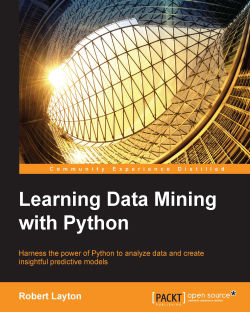### Learning Data Mining with Python4.6 (21 reviews total)
By Robert Layton
• Constantly updated with 100+ new titles each month
• Breadth and depth in over 1,000+ technologies

The next step in the information age is to gain insights from the deluge of data coming our way. Data mining provides a way of finding this insight, and Python is one of the most popular languages for data mining, providing both power and flexibility in analysis.

This book teaches you to design and develop data mining applications using a variety of datasets, starting with basic classification and affinity analysis. Next, we move on to more complex data types including text, images, and graphs. In every chapter, we create models that solve real-world problems.

There is a rich and varied set of libraries available in Python for data mining. This book covers a large number, including the IPython Notebook, pandas, scikit-learn and NLTK.

Each chapter of this book introduces you to new algorithms and techniques. By the end of the book, you will gain a large insight into using Python for data mining, with a good knowledge and understanding of the algorithms and implementations.

Publication date:
July 2015
Publisher
Packt
Pages
344
ISBN
9781784396053

## ChapterÂ 1.Â Getting Started with Data Mining

We are collecting information at a scale that has never been seen before in the history of mankind and placing more day-to-day importance on the use of this information in everyday life. We expect our computers to translate Web pages into other languages, predict the weather, suggest books we would like, and diagnose our health issues. These expectations will grow, both in the number of applications and also in the efficacy we expect. Data mining is a methodology that we can employ to train computers to make decisions with data and forms the backbone of many high-tech systems of today.

The Python language is fast growing in popularity, for a good reason. It gives the programmer a lot of flexibility; it has a large number of modules to perform different tasks; and Python code is usually more readable and concise than in any other languages. There is a large and an active community of researchers, practitioners, and beginners using Python for data mining.

In this chapter, we will introduce data mining with Python. We will cover the following topics:

• What is data mining and where can it be used?

• Setting up a Python-based environment to perform data mining

• An example of affinity analysis, recommending products based on purchasing habits

• An example of (a classic) classification problem, predicting the plant species based on its measurement

## Introducing data mining

Data mining provides a way for a computer to learn how to make decisions with data. This decision could be predicting tomorrow's weather, blocking a spam email from entering your inbox, detecting the language of a website, or finding a new romance on a dating site. There are many different applications of data mining, with new applications being discovered all the time.

Data mining is part of algorithms, statistics, engineering, optimization, and computer science. We also use concepts and knowledge from other fields such as linguistics, neuroscience, or town planning. Applying it effectively usually requires this domain-specific knowledge to be integrated with the algorithms.

Most data mining applications work with the same high-level view, although the details often change quite considerably. We start our data mining process by creating a dataset, describing an aspect of the real world. Datasets comprise of two aspects:

• Samples that are objects in the real world. This can be a book, photograph, animal, person, or any other object.

• Features that are descriptions of the samples in our dataset. Features could be the length, frequency of a given word, number of legs, date it was created, and so on.

The next step is tuning the data mining algorithm. Each data mining algorithm has parameters, either within the algorithm or supplied by the user. This tuning allows the algorithm to learn how to make decisions about the data.

As a simple example, we may wish the computer to be able to categorize people as "short" or "tall". We start by collecting our dataset, which includes the heights of different people and whether they are considered short or tall:

Person

Height

Short or tall?

1

155cm

Short

2

165cm

Short

3

175cm

Tall

4

185cm

Tall

The next step involves tuning our algorithm. As a simple algorithm; if the height is more than x, the person is tall, otherwise they are short. Our training algorithm will then look at the data and decide on a good value for x. For the preceding dataset, a reasonable value would be 170 cm. Anyone taller than 170 cm is considered tall by the algorithm. Anyone else is considered short.

In the preceding dataset, we had an obvious feature type. We wanted to know if people are short or tall, so we collected their heights. This engineering feature is an important problem in data mining. In later chapters, we will discuss methods for choosing good features to collect in your dataset. Ultimately, this step often requires some expert domain knowledge or at least some trial and error.

### Note

In this book, we will introduce data mining through Python. In some cases, we choose clarity of code and workflows, rather than the most optimized way to do this. This sometimes involves skipping some details that can improve the algorithm's speed or effectiveness.

## Using Python and the IPython Notebook

In this section, we will cover installing Python and the environment that we will use for most of the book, the IPython Notebook. Furthermore, we will install the `numpy` module, which we will use for the first set of examples.

### Installing Python

The Python language is a fantastic, versatile, and an easy to use language.

For this book, we will be using Python 3.4, which is available for your system from the Python Organization's website: https://www.python.org/downloads/.

There will be two major versions to choose from, Python 3.4 and Python 2.7. Remember to download and install Python 3.4, which is the version tested throughout this book.

In this book, we will be assuming that you have some knowledge of programming and Python itself. You do not need to be an expert with Python to complete this book, although a good level of knowledge will help.

If you do not have any experience with programming, I recommend that you pick up the Learning Python book from.

The Python organization also maintains a list of two online tutorials for those new to Python:

• For nonprogrammers who want to learn programming through the Python language: https://wiki.python.org/moin/BeginnersGuide/NonProgrammers

• For programmers who already know how to program, but need to learn Python specifically: https://wiki.python.org/moin/BeginnersGuide/Programmers

### Note

Windows users will need to set an environment variable in order to use Python from the command line. First, find where Python 3 is installed; the default location is `C:\Python34`. Next, enter this command into the command line (cmd program): set the enviornment to `PYTHONPATH=%PYTHONPATH%;C:\Python34`. Remember to change the `C:\Python34` if Python is installed into a different directory.

Once you have Python running on your system, you should be able to open a command prompt and run the following code:

```\$ python3
Python 3.4.0 (default, Apr 11 2014, 13:05:11)
[GCC 4.8.2] on Linux
>>> print("Hello, world!")
Hello, world!
>>> exit()
```

Note that we will be using the dollar sign (\$) to denote that a command is to be typed into the terminal (also called a shell or `cmd` on Windows). You do not need to type this character (or the space that follows it). Just type in the rest of the line and press Enter.

After you have the above `"Hello, world!"` example running, exit the program and move on to installing a more advanced environment to run Python code, the IPython Notebook.

### Note

Python 3.4 will include a program called pip, which is a package manager that helps to install new libraries on your system. You can verify that `pip` is working on your system by running the `\$ pip3 freeze` command, which tells you which packages you have installed on your system.

### Installing IPython

IPython is a platform for Python development that contains a number of tools and environments for running Python and has more features than the standard interpreter. It contains the powerful IPython Notebook, which allows you to write programs in a web browser. It also formats your code, shows output, and allows you to annotate your scripts. It is a great tool for exploring datasets and we will be using it as our main environment for the code in this book.

To install IPython on your computer, you can type the following into a command line prompt (not into Python):

```\$ pip install ipython[all]
```

You will need administrator privileges to install this system-wide. If you do not want to (or can't) make system-wide changes, you can install it for just the current user by running this command:

```\$ pip install --user ipython[all]
```

This will install the IPython package into a user-specific locationâ€”you will be able to use it, but nobody else on your computer can. If you are having difficulty with the installation, check the official documentation for more detailed installation instructions:http://ipython.org/install.html.

With the IPython Notebook installed, you can launch it with the following:

```\$ ipython3 notebook
```

This will do two things. First, it will create an IPython Notebook instance that will run in the command prompt you just used. Second, it will launch your web browser and connect to this instance, allowing you to create a new notebook. It will look something similar to the following screenshot (where home/bob will be replaced by your current working directory):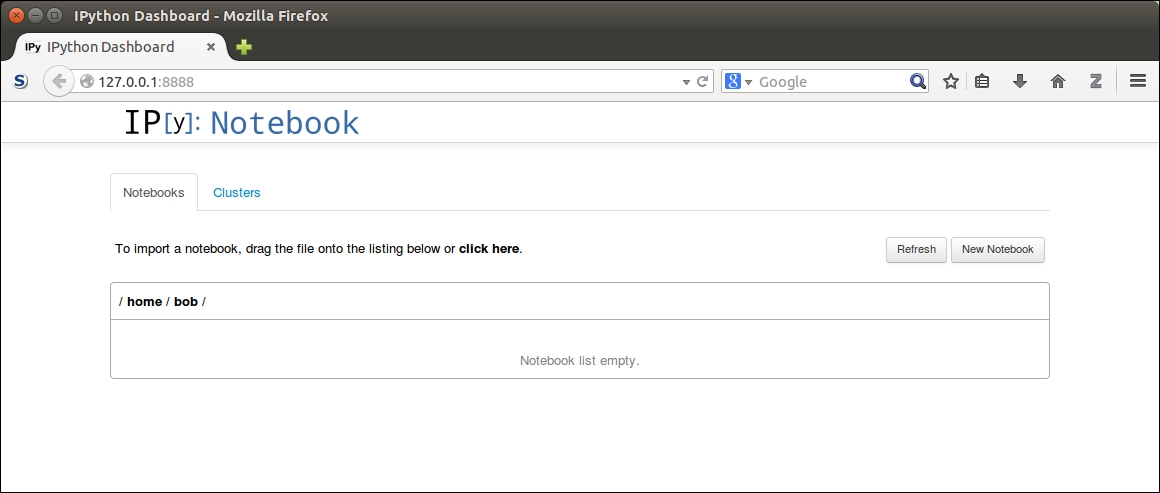To stop the IPython Notebook from running, open the command prompt that has the instance running (the one you used earlier to run the `IPython` command). Then, press Ctrl + C and you will be prompted `Shutdown this notebook server (y/[n])?`. Type y and press Enter and the IPython Notebook will shutdown.

### Installing scikit-learn

The `scikit-learn` package is a machine learning library, written in Python. It contains numerous algorithms, datasets, utilities, and frameworks for performing machine learning. Built upon the scientific python stack, scikit-learn users such as the `numpy` and `scipy` libraries are often optimized for speed. This makes scikit-learn fast and scalable in many instances and also useful for all skill ranges from beginners to advanced research users. We will cover more details of scikit-learn in Chapter 2, Classifying with scikit-learn Estimators.

To install `scikit-learn`, you can use the `pip` utility that comes with Python 3, which will also install the `numpy` and `scipy` libraries if you do not already have them. Open a terminal with administrator/root privileges and enter the following command:

```\$ pip3 install -U scikit-learn
```

### Note

Windows users may need to install the `numpy` and `scipy` libraries before installing scikit-learn. Installation instructions are available at www.scipy.org/install.html for those users.

Users of major Linux distributions such as Ubuntu or Red Hat may wish to install the official package from their package manager. Not all distributions have the latest versions of scikit-learn, so check the version before installing it. The minimum version needed for this book is 0.14.

Those wishing to install the latest version by compiling the source, or view more detailed installation instructions, can go to http://scikit-learn.org/stable/install.html to view the official documentation on installing scikit-learn.

## A simple affinity analysis example

In this section, we jump into our first example. A common use case for data mining is to improve sales by asking a customer who is buying a product if he/she would like another similar product as well. This can be done through affinity analysis, which is the study of when things exist together.

### What is affinity analysis?

Affinity analysis is a type of data mining that gives similarity between samples (objects). This could be the similarity between the following:

• users on a website, in order to provide varied services or targeted advertising

• items to sell to those users, in order to provide recommended movies or products

• human genes, in order to find people that share the same ancestors

We can measure affinity in a number of ways. For instance, we can record how frequently two products are purchased together. We can also record the accuracy of the statement when a person buys object 1 and also when they buy object 2. Other ways to measure affinity include computing the similarity between samples, which we will cover in later chapters.

### Product recommendations

One of the issues with moving a traditional business online, such as commerce, is that tasks that used to be done by humans need to be automated in order for the online business to scale. One example of this is up-selling, or selling an extra item to a customer who is already buying. Automated product recommendations through data mining are one of the driving forces behind the e-commerce revolution that is turning billions of dollars per year into revenue.

In this example, we are going to focus on a basic product recommendation service. We design this based on the following idea: when two items are historically purchased together, they are more likely to be purchased together in the future. This sort of thinking is behind many product recommendation services, in both online and offline businesses.

A very simple algorithm for this type of product recommendation algorithm is to simply find any historical case where a user has brought an item and to recommend other items that the historical user brought. In practice, simple algorithms such as this can do well, at least better than choosing random items to recommend. However, they can be improved upon significantly, which is where data mining comes in.

To simplify the coding, we will consider only two items at a time. As an example, people may buy bread and milk at the same time at the supermarket. In this early example, we wish to find simple rules of the form:

If a person buys product X, then they are likely to purchase product Y

More complex rules involving multiple items will not be covered such as people buying sausages and burgers being more likely to buy tomato sauce.

The dataset can be downloaded from the code package supplied with the book. Download this file and save it on your computer, noting the path to the dataset. For this example, I recommend that you create a new folder on your computer to put your dataset and code in. From here, open your IPython Notebook, navigate to this folder, and create a new notebook.

The dataset we are going to use for this example is a NumPy two-dimensional array, which is a format that underlies most of the examples in the rest of the book. The array looks like a table, with rows representing different samples and columns representing different features.

The cells represent the value of a particular feature of a particular sample. To illustrate, we can load the dataset with the following code:

```import numpy as np
dataset_filename = "affinity_dataset.txt"

For this example, run the IPython Notebook and create an IPython Notebook. Enter the above code into the first cell of your Notebook. You can then run the code by pressing Shift + Enter (which will also add a new cell for the next lot of code). After the code is run, the square brackets to the left-hand side of the first cell will be assigned an incrementing number, letting you know that this cell has been completed. The first cell should look like the following: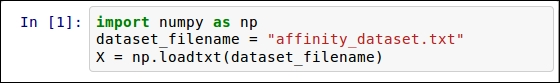For later code that will take more time to run, an asterisk will be placed here to denote that this code is either running or scheduled to be run. This asterisk will be replaced by a number when the code has completed running.

You will need to save the dataset into the same directory as the IPython Notebook. If you choose to store it somewhere else, you will need to change the `dataset_filename` value to the new location.

Next, we can show some of the rows of the dataset to get a sense of what the dataset looks like. Enter the following line of code into the next cell and run it, in order to print the first five lines of the dataset:

`print(X[:5])`

### Tip

You can download the example code files from your account at http://www.packtpub.com for all the Packt Publishing books you have purchased. If you purchased this book elsewhere, you can visit http://www.packtpub.com/support and register to have the files e-mailed directly to you.

The result will show you which items were bought in the first five transactions listed: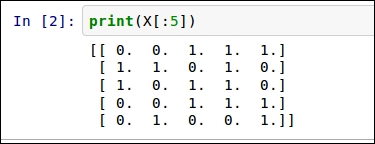The dataset can be read by looking at each row (horizontal line) at a time. The first row `(0, 0, 1, 1, 1)` shows the items purchased in the first transaction. Each column (vertical row) represents each of the items. They are bread, milk, cheese, apples, and bananas, respectively. Therefore, in the first transaction, the person bought cheese, apples, and bananas, but not bread or milk.

Each of these features contain binary values, stating only whether the items were purchased and not how many of them were purchased. A 1 indicates that "at least 1" item was bought of this type, while a 0 indicates that absolutely none of that item was purchased.

### Implementing a simple ranking of rules

We wish to find rules of the type If a person buys product X, then they are likely to purchase product Y. We can quite easily create a list of all of the rules in our dataset by simply finding all occasions when two products were purchased together. However, we then need a way to determine good rules from bad ones. This will allow us to choose specific products to recommend.

Rules of this type can be measured in many ways, of which we will focus on two: support and confidence.

Support is the number of times that a rule occurs in a dataset, which is computed by simply counting the number of samples that the rule is valid for. It can sometimes be normalized by dividing by the total number of times the premise of the rule is valid, but we will simply count the total for this implementation.

While the support measures how often a rule exists, confidence measures how accurate they are when they can be used. It can be computed by determining the percentage of times the rule applies when the premise applies. We first count how many times a rule applies in our dataset and divide it by the number of samples where the premise (the `if` statement) occurs.

As an example, we will compute the support and confidence for the rule if a person buys apples, they also buy bananas.

As the following example shows, we can tell whether someone bought apples in a transaction by checking the value of `sample`, where a sample is assigned to a row of our matrix: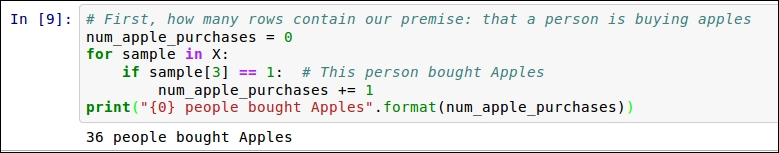Similarly, we can check if bananas were bought in a transaction by seeing if the value for `sample` is equal to 1 (and so on). We can now compute the number of times our rule exists in our dataset and, from that, the confidence and support.

Now we need to compute these statistics for all rules in our database. We will do this by creating a dictionary for both valid rules and invalid rules. The key to this dictionary will be a tuple (premise and conclusion). We will store the indices, rather than the actual feature names. Therefore, we would store (3 and 4) to signify the previous rule If a person buys Apples, they will also buy Bananas. If the premise and conclusion are given, the rule is considered valid. While if the premise is given but the conclusion is not, the rule is considered invalid for that sample.

To compute the confidence and support for all possible rules, we first set up some dictionaries to store the results. We will use `defaultdict` for this, which sets a default value if a key is accessed that doesn't yet exist. We record the number of valid rules, invalid rules, and occurrences of each premise:

```from collections import defaultdict
valid_rules = defaultdict(int)
invalid_rules = defaultdict(int)
num_occurances = defaultdict(int)```

Next we compute these values in a large loop. We iterate over each sample and feature in our dataset. This first feature forms the premise of the ruleâ€”if a person buys a product premise:

```for sample in X:
for premise in range(4):```

We check whether the premise exists for this sample. If not, we do not have any more processing to do on this sample/premise combination, and move to the next iteration of the loop:

`  if sample[premise] == 0: continue`

If the premise is valid for this sample (it has a value of 1), then we record this and check each conclusion of our rule. We skip over any conclusion that is the same as the premiseâ€”this would give us rules such as If a person buys Apples, then they buy Apples, which obviously doesn't help us much;

```  num_occurances[premise] += 1
for conclusion in range(n_features):
if premise == conclusion: continue```

If the conclusion exists for this sample, we increment our valid count for this rule. If not, we increment our invalid count for this rule:

```  if sample[conclusion] == 1:
valid_rules[(premise, conclusion)] += 1
else:
invalid_rules[(premise, conclusion)] += 1
```

We have now completed computing the necessary statistics and can now compute the support and confidence for each rule. As before, the support is simply our `valid_rules` value:

`support = valid_rules`

The confidence is computed in the same way, but we must loop over each rule to compute this:

```confidence = defaultdict(float)
for premise, conclusion in valid_rules.keys():
rule = (premise, conclusion)
confidence[rule] = valid_rules[rule] / num_occurances[premise]```

We now have a dictionary with the support and confidence for each rule. We can create a function that will print out the rules in a readable format. The signature of the rule takes the premise and conclusion indices, the support and confidence dictionaries we just computed, and the features array that tells us what the features mean:

```def print_rule(premise, conclusion,
support, confidence, features):```

We get the names of the features for the premise and conclusion and print out the rule in a readable format:

```    premise_name = features[premise]
conclusion_name = features[conclusion]
{1}".format(premise_name, conclusion_name))
```

Then we print out the `Support` and `Confidence` of this rule:

```    print(" - Support: {0}".format(support[(premise,
conclusion)]))
print(" - Confidence: {0:.3f}".format(confidence[(premise,
conclusion)]))```

We can test the code by calling it in the following wayâ€”feel free to experiment with different premises and conclusions: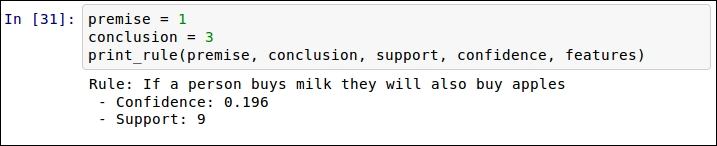### Ranking to find the best rules

Now that we can compute the support and confidence of all rules, we want to be able to find the best rules. To do this, we perform a ranking and print the ones with the highest values. We can do this for both the support and confidence values.

To find the rules with the highest support, we first sort the support dictionary. Dictionaries do not support ordering by default; the `items()` function gives us a list containing the data in the dictionary. We can sort this list using the `itemgetter` class as our key, which allows for the sorting of nested lists such as this one. Using `itemgetter(1)` allows us to sort based on the values. `Setting reverse=True` gives us the highest values first:

```from operator import itemgetter
sorted_support = sorted(support.items(), key=itemgetter(1), reverse=True)```

We can then print out the top five rules:

```for index in range(5):
print("Rule #{0}".format(index + 1))
premise, conclusion = sorted_support[index]
print_rule(premise, conclusion, support, confidence, features)```

The result will look like the following: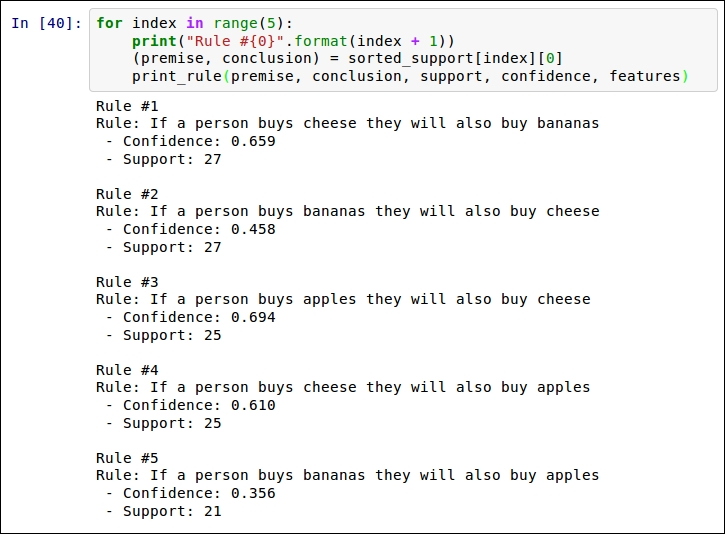Similarly, we can print the top rules based on confidence. First, compute the sorted confidence list:

`sorted_confidence = sorted(confidence.items(), key=itemgetter(1), reverse=True)`

Next, print them out using the same method as before. Note the change to `sorted_confidence` on the third line;

```for index in range(5):
print("Rule #{0}".format(index + 1))
premise, conclusion = sorted_confidence[index]
print_rule(premise, conclusion, support, confidence, features)```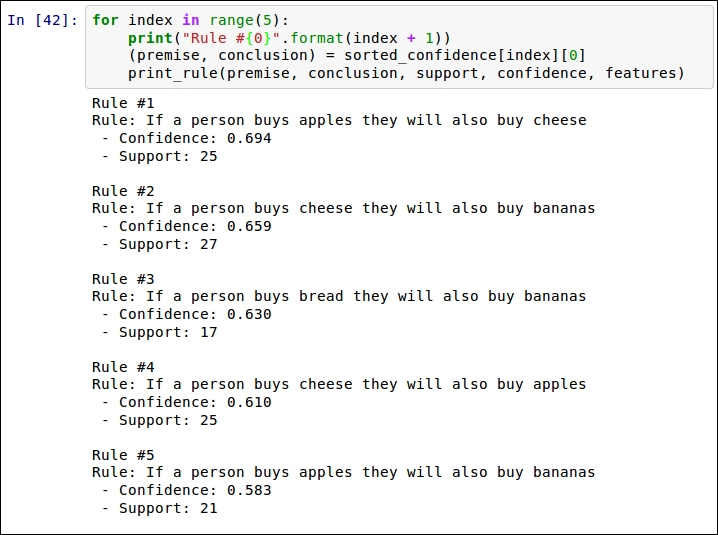Two rules are near the top of both lists. The first is If a person buys apples, they will also buy cheese, and the second is If a person buys cheese, they will also buy bananas. A store manager can use rules like these to organize their store. For example, if apples are on sale this week, put a display of cheeses nearby. Similarly, it would make little sense to put both bananas on sale at the same time as cheese, as nearly 66 percent of people buying cheese will buy bananas anywayâ€”our sale won't increase banana purchases all that much.

Data mining has great exploratory power in examples like this. A person can use data mining techniques to explore relationships within their datasets to find new insights. In the next section, we will use data mining for a different purpose: prediction.

## A simple classification example

In the affinity analysis example, we looked for correlations between different variables in our dataset. In classification, we instead have a single variable that we are interested in and that we call the class (also called the target). If, in the previous example, we were interested in how to make people buy more apples, we could set that variable to be the class and look for classification rules that obtain that goal. We would then look only for rules that relate to that goal.

## What is classification?

Classification is one of the largest uses of data mining, both in practical use and in research. As before, we have a set of samples that represents objects or things we are interested in classifying. We also have a new array, the class values. These class values give us a categorization of the samples. Some examples are as follows:

• Determining the species of a plant by looking at its measurements. The class value here would be Which species is this?.

• Determining if an image contains a dog. The class would be Is there a dog in this image?.

• Determining if a patient has cancer based on the test results. The class would be Does this patient have cancer?.

While many of the examples above are binary (yes/no) questions, they do not have to be, as in the case of plant species classification in this section.

The goal of classification applications is to train a model on a set of samples with known classes, and then apply that model to new unseen samples with unknown classes. For example, we want to train a spam classifier on my past e-mails, which I have labeled as spam or not spam. I then want to use that classifier to determine whether my next email is spam, without me needing to classify it myself.

The dataset we are going to use for this example is the famous Iris database of plant classification. In this dataset, we have 150 plant samples and four measurements of each: sepal length, sepal width, petal length, and petal width (all in centimeters). This classic dataset (first used in 1936!) is one of the classic datasets for data mining. There are three classes: Iris Setosa, Iris Versicolour, and Iris Virginica. The aim is to determine which type of plant a sample is, by examining its measurements.

The `scikit-learn` library contains this dataset built-in, making the loading of the dataset straightforward:

```from sklearn.datasets import load_iris
X = dataset.data
y = dataset.target```

You can also print(`dataset.DESCR`) to see an outline of the dataset, including some details about the features.

The features in this dataset are continuous values, meaning they can take any range of values. Measurements are a good example of this type of feature, where a measurement can take the value of 1, 1.2, or 1.25 and so on. Another aspect about continuous features is that feature values that are close to each other indicate similarity. A plant with a sepal length of 1.2 cm is like a plant with sepal width of 1.25 cm.

In contrast are categorical features. These features, while often represented as numbers, cannot be compared in the same way. In the Iris dataset, the class values are an example of a categorical feature. The class 0 represents Iris Setosa, class 1 represents Iris Versicolour, and class 2 represents Iris Virginica. This doesn't mean that Iris Setosa is more similar to Iris Versicolour than it is to Iris Virginicaâ€”despite the class value being more similar. The numbers here represent categories. All we can say is whether categories are the same or different.

There are other types of features too, some of which will be covered in later chapters.

While the features in this dataset are continuous, the algorithm we will use in this example requires categorical features. Turning a continuous feature into a categorical feature is a process called discretization.

A simple discretization algorithm is to choose some threshold and any values below this threshold are given a value 0. Meanwhile any above this are given the value 1. For our threshold, we will compute the mean (average) value for that feature. To start with, we compute the mean for each feature:

```attribute_means = X.mean(axis=0)
```

This will give us an array of length 4, which is the number of features we have. The first value is the mean of the values for the first feature and so on. Next, we use this to transform our dataset from one with continuous features to one with discrete categorical features:

`X_d = np.array(X >= attribute_means, dtype='int')`

We will use this new `X_d` dataset (for X discretized) for our training and testing, rather than the original dataset (X).

### Implementing the OneR algorithm

OneR is a simple algorithm that simply predicts the class of a sample by finding the most frequent class for the feature values. OneR is a shorthand for One Rule, indicating we only use a single rule for this classification by choosing the feature with the best performance. While some of the later algorithms are significantly more complex, this simple algorithm has been shown to have good performance in a number of real-world datasets.

The algorithm starts by iterating over every value of every feature. For that value, count the number of samples from each class that have that feature value. Record the most frequent class for the feature value, and the error of that prediction.

For example, if a feature has two values, 0 and 1, we first check all samples that have the value 0. For that value, we may have 20 in class A, 60 in class B, and a further 20 in class C. The most frequent class for this value is B, and there are 40 instances that have difference classes. The prediction for this feature value is B with an error of 40, as there are 40 samples that have a different class from the prediction. We then do the same procedure for the value 1 for this feature, and then for all other feature value combinations.

Once all of these combinations are computed, we compute the error for each feature by summing up the errors for all values for that feature. The feature with the lowest total error is chosen as the One Rule and then used to classify other instances.

In code, we will first create a function that computes the class prediction and error for a specific feature value. We have two necessary imports, `defaultdict` and `itemgetter`, that we used in earlier code:

```from collections import defaultdict
from operator import itemgetter```

Next, we create the function definition, which needs the dataset, classes, the index of the feature we are interested in, and the value we are computing:

`def train_feature_value(X, y_true, feature_index, value):`

We then iterate over all the samples in our dataset, counting the actual classes for each sample with that feature value:

```    class_counts = defaultdict(int)
for sample, y in zip(X, y_true):
if sample[feature_index] == value:
class_counts[y] += 1```

We then find the most frequently assigned class by sorting the `class_counts` dictionary and finding the highest value:

`    sorted_class_counts = sorted(class_counts.items(), key=itemgetter(1), reverse=True) most_frequent_class = sorted_class_counts`

Finally, we compute the error of this rule. In the OneR algorithm, any sample with this feature value would be predicted as being the most frequent class. Therefore, we compute the error by summing up the counts for the other classes (not the most frequent). These represent training samples that this rule does not work on:

```incorrect_predictions = [class_count for class_value, class_count
in class_counts.items()
if class_value != most_frequent_class]
error = sum(incorrect_predictions)
```

Finally, we return both the predicted class for this feature value and the number of incorrectly classified training samples, the error, of this rule:

`    return most_frequent_class, error`

With this function, we can now compute the error for an entire feature by looping over all the values for that feature, summing the errors, and recording the predicted classes for each value.

The function header needs the dataset, classes, and feature index we are interested in:

`def train_on_feature(X, y_true, feature_index):`

Next, we find all of the unique values that the given feature takes. The indexing in the next line looks at the whole column for the given feature and returns it as an array. We then use the set function to find only the unique values:

`    values = set(X[:,feature_index])`

Next, we create our dictionary that will store the predictors. This dictionary will have feature values as the keys and classification as the value. An entry with key 1.5 and value 2 would mean that, when the feature has value set to 1.5, classify it as belonging to class 2. We also create a list storing the errors for each feature value:

```    predictors = {}
errors = []```

As the main section of this function, we iterate over all the unique values for this feature and use our previously defined `train_feature_value()` function to find the most frequent class and the error for a given feature value. We store the results as outlined above:

```    for current_value in values:
most_frequent_class, error = train_feature_value(X, y_true, feature_index, current_value)
predictors[current_value] = most_frequent_class
errors.append(error)```

Finally, we compute the total errors of this rule and return the predictors along with this value:

```    total_error = sum(errors)
return predictors, total_error```

### Testing the algorithm

When we evaluated the affinity analysis algorithm of the last section, our aim was to explore the current dataset. With this classification, our problem is different. We want to build a model that will allow us to classify previously unseen samples by comparing them to what we know about the problem.

For this reason, we split our machine-learning workflow into two stages: training and testing. In training, we take a portion of the dataset and create our model. In testing, we apply that model and evaluate how effectively it worked on the dataset. As our goal is to create a model that is able to classify previously unseen samples, we cannot use our testing data for training the model. If we do, we run the risk of overfitting.

Overfitting is the problem of creating a model that classifies our training dataset very well, but performs poorly on new samples. The solution is quite simple: never use training data to test your algorithm. This simple rule has some complex variants, which we will cover in later chapters; but, for now, we can evaluate our OneR implementation by simply splitting our dataset into two small datasets: a training one and a testing one. This workflow is given in this section.

The `scikit-learn` library contains a function to split data into training and testing components:

`from sklearn.cross_validation import train_test_split`

This function will split the dataset into two subdatasets, according to a given ratio (which by default uses 25 percent of the dataset for testing). It does this randomly, which improves the confidence that the algorithm is being appropriately tested:

`Xd_train, Xd_test, y_train, y_test = train_test_split(X_d, y, random_state=14)`

We now have two smaller datasets: `Xd_train` contains our data for training and `Xd_test` contains our data for testing. `y_train` and `y_test` give the corresponding class values for these datasets.

We also specify a specific `random_state`. Setting the random state will give the same split every time the same value is entered. It will look random, but the algorithm used is deterministic and the output will be consistent. For this book, I recommend setting the random state to the same value that I do, as it will give you the same results that I get, allowing you to verify your results. To get truly random results that change every time you run it, set `random_state` to `none`.

Next, we compute the predictors for all the features for our dataset. Remember to only use the training data for this process. We iterate over all the features in the dataset and use our previously defined functions to train the predictors and compute the errors:

```all_predictors = {}
errors = {}
for feature_index in range(Xd_train.shape):
predictors, total_error = train_on_feature(Xd_train, y_train, feature_index)
all_predictors[feature_index] = predictors
errors[feature_index] = total_error```

Next, we find the best feature to use as our "One Rule", by finding the feature with the lowest error:

`best_feature, best_error = sorted(errors.items(), key=itemgetter(1))`

We then create our `model` by storing the predictors for the best feature:

```model = {'feature': best_feature,
'predictor': all_predictors[best_feature]}```

Our model is a dictionary that tells us which feature to use for our One Rule and the predictions that are made based on the values it has. Given this model, we can predict the class of a previously unseen sample by finding the value of the specific feature and using the appropriate predictor. The following code does this for a given sample:

```variable = model['variable']
predictor = model['predictor']
prediction = predictor[int(sample[variable])]```

Often we want to predict a number of new samples at one time, which we can do using the following function; we use the above code, but iterate over all the samples in a dataset, obtaining the prediction for each sample:

```def predict(X_test, model):
variable = model['variable']
predictor = model['predictor']
y_predicted = np.array([predictor[int(sample[variable])] for sample in X_test])
return y_predicted```

For our `testing` dataset, we get the predictions by calling the following function:

`y_predicted = predict(X_test, model)`

We can then compute the accuracy of this by comparing it to the known classes:

```accuracy = np.mean(y_predicted == y_test) * 100
print("The test accuracy is {:.1f}%".format(accuracy))```

This gives an accuracy of 68 percent, which is not bad for a single rule!

## Summary

In this chapter, we introduced data mining using Python. If you were able to run the code in this section (note that the full code is available in the supplied code package), then your computer is set up for much of the rest of the book. Other Python libraries will be introduced in later chapters to perform more specialized tasks.

We used the IPython Notebook to run our code, which allows us to immediately view the results of a small section of the code. This is a useful framework that will be used throughout the book.

We introduced a simple affinity analysis, finding products that are purchased together. This type of exploratory analysis gives an insight into a business process, an environment, or a scenario. The information from these types of analysis can assist in business processes, finding the next big medical breakthrough, or creating the next artificial intelligence.

Also, in this chapter, there was a simple classification example using the OneR algorithm. This simple algorithm simply finds the best feature and predicts the class that most frequently had this value in the training dataset.

Over the next few chapters, we will expand on the concepts of classification and affinity analysis. We will also introduce the scikit-learn package and the algorithms it includes.

• ##### Robert Layton

Robert Layton is a data scientist investigating data-driven applications to businesses across a number of sectors. He received a PhD investigating cybercrime analytics from the Internet Commerce Security Laboratory at Federation University Australia, before moving into industry, starting his own data analytics company dataPipeline. Next, he created Eureaktive, which works with tech-based startups on developing their proof-of-concepts and early-stage prototypes. Robert also runs the LearningTensorFlow website, which is one of the world's premier tutorial websites for Google's TensorFlow library.

Robert is an active member of the Python community, having used Python for more than 8 years. He has presented at PyConAU for the last four years and works with Python Charmers to provide Python-based training for businesses and professionals from a wide range of organisations.

Robert can be best reached via Twitter @robertlayton

Browse publications by this author

### Latest Reviews

(21 reviews total)